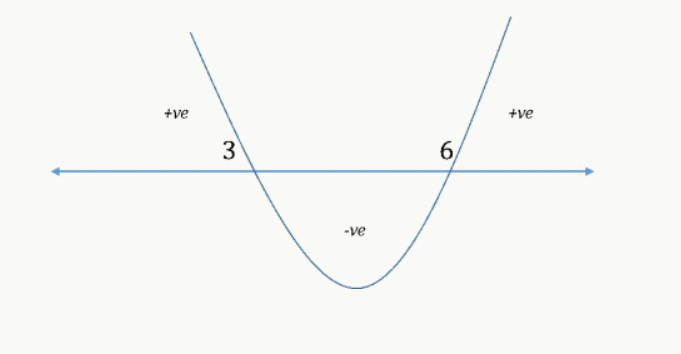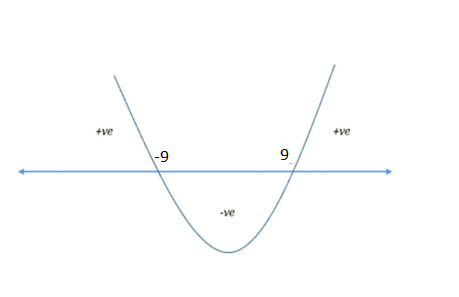GMAT Question of the Day: Daily via email | Daily via Instagram New to GMAT Club? Watch this Video

 It is currently 29 May 2020, 00:24### GMAT Club Daily Prep

#### Thank you for using the timer - this advanced tool can estimate your performance and suggest more practice questions. We have subscribed you to Daily Prep Questions via email.

Customized
for You

we will pick new questions that match your level based on your Timer History

Track

every week, we’ll send you an estimated GMAT score based on your performance

Practice
Pays

we will pick new questions that match your level based on your Timer History

#### Not interested in getting valuable practice questions and articles delivered to your email? No problem, unsubscribe here.# Simple yet Effective Approach to Solve Quadratic Inequalities

Author Message
TAGS:

### Hide Tags

MBA Section DirectorV
Affiliations: GMAT Club
Joined: 21 Feb 2012
Posts: 7603
City: Pune

### Show Tags

GMAT Tip of the Week: Simple yet Effective Approach to Solve Quadratic Inequalities

This topic is a part of the GMAT Club Tip of the Week Series

Objective of the article

Algebra is one of the most important area to score beyond Q49 on GMAT. Learning Algebra is a pre-requisite to master other sections such as Number Properties, Word Problems and Geometry. In this article, we would help you a simple yet effective approach to solve questions on one of the most confusing topics in Algebra – Quadratic Inequalities.

We suggest that you follow the sequence mentioned below:

1. Get started by going through the video lessons embedded above to learn the basics.
2. Then go through the following questions in this article to learn how to solve Quadratic Inequalities related questions.

Example 1

Find the range of values of x satisfying the inequality (x – 3) * (x – 6) < 0?

A. 3 < x < 6
B. x < 3 or x > 6
C. 0 < x < 6
D. 3 ≤ x ≤ 6
E. x ≤ 3 or x ≥ 6

Solution

As taught in the concept video, we can draw a wavy-line to solve this inequality

• For that, we need to find the zero points of this inequality, they are 3 and 6.
• Now, plot these points on a number line, and draw a wavy line starting from the right top region and passing through the zero points, 6 and 3.
• So, the wavy-line will be as follows:Now, let’s focus our energy on drawing inferences from this diagram:

1. The expression will be positive for the range of values for which the curve is above the number line.
a. In the above example, the pertinent ranges are x < 3 and x > 6. So, any value of x either less than 3 or greater than 6 will make the above expression positive in sign.

2. The expression will be equal to zero for the values at which the curve intersects the number line.
a. In the above example, the pertinent points are x = 3 and x = 6. So, both these values make the expression zero.

3. The expression will be negative for the range of values for which the curve is below the number line.
a. In the above example, there is only one portion where the curve is below the number line and this portion corresponds to the range 3 < x < 6. So, any value of x strictly between 3 and 6 will make the above expression negative in sign.

Since, the above question asks for the range of values of x for which the expression is negative, the answer would be 3 < x < 6.

Hence, the correct answer is Option A.

Key Takeaways

1. In this question, we learnt how to solve a simple quadratic inequality using wavy-line method.
a) And, to do that, we always start off with finding the zero points, and plotting them on a number line, which divides the number line into multiple regions.
b) Then, we can draw a wavy-line starting from the right-top region, such that it passes through all the zero points present on the number line.

2. We also learnt that the zero points must not be included in the answer, if the given expression is not equal to zero.

With these learnings in mind, let us now move to the next question.

Example 2

If x is an integer, then how many values of x, satisfy the inequality, $$x^2 ≥ 81$$?

A. 9
B. 10
C. 17
D. 19
E. Cannot be determined

Solution

If we observe this question is not in the standard form of a quadratic inequality.

So, as we have seen in the concept video, we need to make the RHS of the inequality 0, to bring the above inequality to the standard form.
• For that, we need to subtract 81 on both sides
o Thus, we get, $$x^2 – 81 ≥ 0$$, which is in the standard form of a quadratic inequality.

• Now, we can write, $$x^2 – 81$$ as $$x^2 - 9^2$$, which is in the form of $$a^2 – b^2$$
• Thus, $$x^2 – 81 ≥ 0$$ can be written as (x + 9) * (x - 9) ≥ 0, since, $$a^2 – b^2 = (a + b) * (a - b)$$

Let’s now use our learning from previous question and solve this question.

First, we need to find out the zero points and then draw the wavy-line by plotting these points on a number line.

So, the zero points of this inequality are -9 and 9, and the wavy-like will be as shown below:Now, let’s focus our energy on drawing inferences from this:

1. The expression will be positive for the range of values for which the curve is above the number line.
a. In the above example, the pertinent ranges are x < -9 and x > 9. So, any value of x either less than -9 or greater than 9 will make the above expression positive in sign.

2. The expression will be equal to zero for the values at which the curve intersects the number line.
a. In the above example, the pertinent points are x = -9 and x = 9. So, both these values make the expression zero.

3. The expression will be negative for the range of values for which the curve is below the number line.
a. In the above example, there is only one portion where the curve is below the number line and this portion corresponds to the range -9 < x < 9. So, any value of x strictly between -9 and 9 will make the above expression negative in sign.

Since, the above question asks for the range of values of x for which the expression is non-negative zero, the answer would be x ≤ -9 or x ≥ 9.

Note: We are including the equality, since the inequality includes 0.

Therefore, we cannot determine the number of integer values of x that satisfy the given inequality, since the range is open, and x can take infinite number of values.

Hence, the correct answer is Option E.

Key Takeaways

1. How to get a quadratic inequality to its standard form, $$ax^2 + bx + c$$ < or > d, where a is positive and d is zero.
2. How to use the constraint to find an answer.

Get amazing free resources

e-GMAT offers a variety of free video lessons (25+) and diagnostic quizzes (400+ questions) on different topics. Start your free trial and learn using our immersive course.

_________________
MBA Spotlight - GMAT Club's Top 20 MBA Fair.
2020 MBA Applicants: Introduce Yourself Here!
Non-Human UserJoined: 09 Sep 2013
Posts: 15011

### Show Tags

Hello from the GMAT Club BumpBot!

Thanks to another GMAT Club member, I have just discovered this valuable topic, yet it had no discussion for over a year. I am now bumping it up - doing my job. I think you may find it valuable (esp those replies with Kudos).

Want to see all other topics I dig out? Follow me (click follow button on profile). You will receive a summary of all topics I bump in your profile area as well as via email.
_________________Re: Simple yet Effective Approach to Solve Quadratic Inequalities   [#permalink] 24 Feb 2020, 21:41

# Simple yet Effective Approach to Solve Quadratic Inequalities